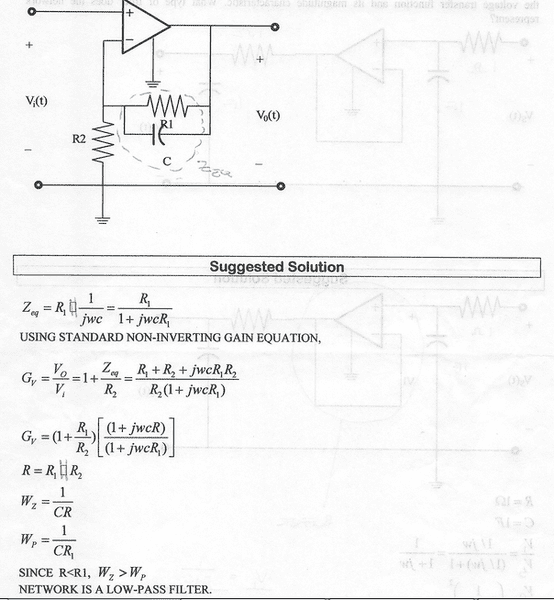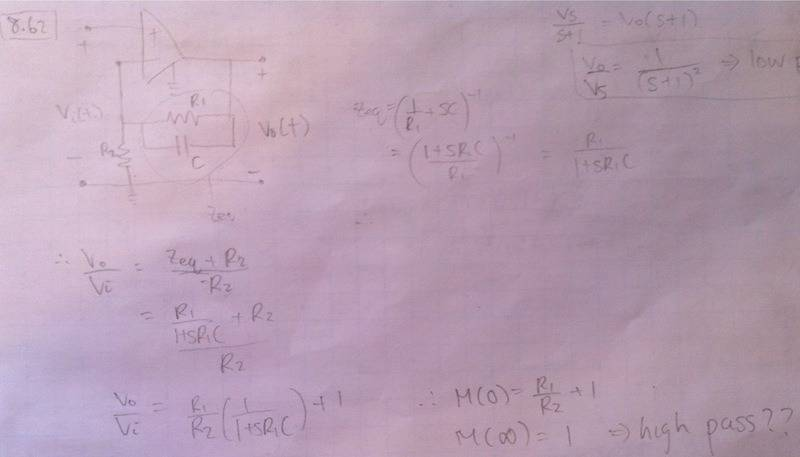# Is this a high pass or low pass active filter?

## Homework Statement

Here is the problem with the solution:## The Attempt at a Solution

I don't understand why the filter is low pass. How I solve this usually is I plug in ω=0 and ω=∞. When I plug in 0 I get the gain of a non inverting op amp. When I plug in ∞ I get that the transfer function (gain) is equal to 1. Since it is equal to 1 doesn't that mean it lets high frequencies pass? I remember in passive filters when you get 1 for ω=∞ it means it is a high pass filter. HOWEVER, the tricky thing about active filters is that when ω=0, the gain is not 0, instead it is 1+R1/R2 which I think is why I am confused.

berkeman
Mentor

## Homework Statement

Here is the problem with the solution:

## The Attempt at a Solution

I don't understand why the filter is low pass. How I solve this usually is I plug in ω=0 and ω=∞. When I plug in 0 I get the gain of a non inverting op amp. When I plug in ∞ I get that the transfer function (gain) is equal to 1. Since it is equal to 1 doesn't that mean it lets high frequencies pass? I remember in passive filters when you get 1 for ω=∞ it means it is a high pass filter. HOWEVER, the tricky thing about active filters is that when ω=0, the gain is not 0, instead it is 1+R1/R2 which I think is why I am confused.

That gain equation looks wrong. First of all, it's an inverting amp, so the gain has to be negative. Second, the feedback impedance goes to zero at high frequencies, so the gain should go toward -0 at high frequencies...

That gain equation looks wrong. First of all, it's an inverting amp, so the gain has to be negative. Second, the feedback impedance goes to zero at high frequencies, so the gain should go toward -0 at high frequencies...

Hi,

I compared it with wikipedia and it looks like a non inverting amp to me? :S

http://en.wikipedia.org/wiki/Operational_amplifier#Non-inverting_amplifier

I also solved it on my own, as you can see, when I plug in a high frequency I do not get 0. Instead I get 1.That gain equation looks wrong. First of all, it's an inverting amp, so the gain has to be negative.

Have another look.. it is not inverting :) Vi is applied to the + t-erminal and will also appear at the - terminal. This causes current to flow from the top of R2 to ground, so the op amp will be pushing current from the output toward ground through R2 and R1||C, making the output at higher voltage than the voltage at the - terminal which is Vi. So Vo > Vi.

thebeast, what you did was right. You just need to be more careful about your conclusion.

At w->0, the capacitor has infinite impedance so it is out of the circuit. The output is Vo = (1+R1/(R1+R2))*Vi.

At w->∞, the capacitor is a short so R1 is out of the circuit. Now Vo=Vi.

The output at low frequencies is higher than the output at high frequencies so this is a low pass filter. It's a little different because the gain does not approach 0 as w→∞

You can also see this from the suggested solution. There is both a zero and a pole. The pole occurs at a lower frequency so a bode plot will show the gain flat, then head down at -20db/decade at the low pass corner frequency (the pole) and then become constant when the high pass corner frequency is met (the zero adds a rising slope of 20db/decade). This is what you found -- higher gain at 0 and lower but non-zero gain at infinity.

Have another look.. it is not inverting :) Vi is applied to the + t-erminal and will also appear at the - terminal. This causes current to flow from the top of R2 to ground, so the op amp will be pushing current from the output toward ground through R2 and R1||C, making the output at higher voltage than the voltage at the - terminal which is Vi. So Vo > Vi.

thebeast, what you did was right. You just need to be more careful.

At w->0, the capacitor has infinite impedance so it is out of the circuit. The output is Vo = (1+R1/(R1+R2))*Vi.

At w->∞, the capacitor is a short so R1 is out of the circuit. Now Vo=Vi.

The output at low frequencies is higher than the output at high frequencies so this is a low pass filter. It's a little different because the gain does not approach 0 as w→∞

You can also see this from the suggested solution. There is both a zero and a pole. The zero occurs at a lower frequency so a bode plot will show the gain flat, then head down at -20db/decade at the low pass corner frequency (the zero) and then stay constant when the high pass corner frequency is met (the pole adds a rising slope of 20db/decade). This is what you found -- higher gain at 0 and lower but non-zero gain at infinity.

Thank you! Your first paragraph really helped me in understanding how the non inverting op amp works.

Also... So whichever one is higher is the frequency that is allowed to pass? Unfortunately our professor did not teach us bode plots so I think that is the reason to why I am having difficulty in understanding this. I will definitely check it out though!

berkeman
Mentor
Have another look.. it is not inverting :)

Oops! You are correct, my mistake. I usually draw my opamp circuits with the inverting terminal on top, but that's still not a good excuse for missing the non-inverting configuration.

Unfortunately you quoted me before I finished an edit :) I had zero and pole swapped in my last paragraph. My post is corrected but the quoted text still has it wrong.

Unfortunately our professor did not teach us bode plots so I think that is the reason to why I am having difficulty in understanding this. I will definitely check it out though!

Alright well the solution is depending on a bode plot argument so that may be a problem for you to follow.

Also... So whichever one is higher is the frequency that is allowed to pass?

All frequencies are allowed to pass since there is no w where the gain is zero; it's just that some frequencies are emphasized and some are not, making them smaller in comparison. You found the low frequencies get high gain and the high frequencies get (relatively) low gain.